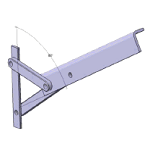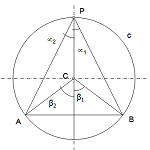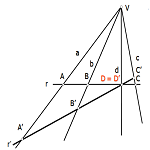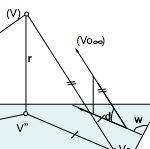# Drawing

## Learning method for engineering drawing

The following links are part of the materials for active learning methodology Technical Drawing and Geometry. These materials are necessary to develop own technical activity of engineering and other scientific nature.

The geometric idealization applies both in the description of models such as the calculation and analysis of complex functions. Geometry is the structural basis on which these techniques are basedGeometric Logical Method (MLG) A streamlined way to analyze the degrees of freedom of a geometric model.Metric Geometry Introduction to Classical Geometry, with a simplified construction sequence, simple models based knowledge structuring. Intensively to structure a geometry that allows complex models.Projective Geometry (GP)Projection operations and allow you to set, together with the definition of projective invariants (Triples and quadruples) an abstract model of reasoning application in large systems of linear perspective and representation.Representation Systems (SR)Representation systems are based on the operations or projective transformations. They use these concepts with simple rules for representation, on a plane, three dimensional models of.